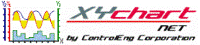XY Chart NET 3 Control Reference
YStack Property

This property is only applicable if YStacking is set to TRUE.

Use this property to return/set values that determines the stacking characteristics for a specific y-stack.  YStack uses the YStack Class set of properties for its characteristics.

Syntax
 [Visual Basic] Property YStack(YStackNumber As Integer) As C_YStack [C#] XYChartNet.XYChartNETCtl.C_YStack XYChartNETCtl.get_YStack(int YStackNumber) [C++] property XYChartNet::XYChartNETCtl::C_YStack ^ XYChartNet::XYChartNETCtl::YStack[int]

Parameters
YStackNumber
The index of the y-stack graph to set/get.  Valid values are 0..(NumYStacks - 1).
Example
```' XYChartNETCtl1 is the name of the XY Chart NET control instance placed on the form.

Dim bGraphStacked As Boolean

With XYChartNETCtl1
.NumProfiles = 10                  'Set the number of profiles to plot to 10
.NumYScales = 8                    'Set the number of y-axis scales to 8
.YStacking = True                  'Stack the graphs
.NumYStacks = 5                    'Set the number of y-stacks to 5

bGraphStacked = .YStack(0).Visible 'Determine if y-stack 0 is displayed

.YStack(0).Visible = True          'Display the graph
.YStack(1).Visible = True          'Display the graph
.YStack(2).Visible = False         'Do not display the third stacked graph
.YStack(3).Visible = True          'Display the graph
.YStack(4).Visible = True          'Display the graph

.YStack(0).HeightPercentage = 10   'Display graph 0 to use 10% of the overall graph height
.YStack(1).HeightPercentage = 20   'Display graph 1 to use 20% of the overall graph height
.YStack(2).HeightPercentage = 25   'Display graph 2 to use 25% of the overall graph height
.YStack(3).HeightPercentage = 15   'Display graph 3 to use 15% of the overall graph height
.YStack(4).HeightPercentage = 30   'Display graph 4 to use 30% of the overall graph height

.YStack(0).NumYScales = 1          'Display y-scale 0 on graph 0
.YStack(1).NumYScales = 1          'Display y-scale 1 on graph 1
.YStack(2).NumYScales = 2          'Display y-scales 2 and 3 on graph 2
.YStack(3).NumYScales = 3          'Display y-scales 4, 5 and 6 on graph 3
.YStack(4).NumYScales = 1          'Display y-scale 7 on graph 4

.Refresh                           'Refresh required to update chart with changes made
End With
```
```// XYChartNETCtl1 is the name of the XY Chart NET control instance placed on the form.

XYChartNETCtl1.NumProfiles = 10;                     //Set the number of profiles to plot to 10
XYChartNETCtl1.NumYScales = 8;                       //Set the number of y-axis scales to 8
XYChartNETCtl1.YStacking = true;                     //Stack the graphs
XYChartNETCtl1.NumYStacks = 5;                       //Set the number of y-stacks to 5

XYChartNETCtl1.get_YStack(0).HeightPercentage = 10;  //Display graph 0 to use 10% of the overall graph height
XYChartNETCtl1.get_YStack(1).HeightPercentage = 20;  //Display graph 1 to use 20% of the overall graph height
XYChartNETCtl1.get_YStack(2).HeightPercentage = 25;  //Display graph 2 to use 25% of the overall graph height
XYChartNETCtl1.get_YStack(3).HeightPercentage = 15;  //Display graph 3 to use 15% of the overall graph height
XYChartNETCtl1.get_YStack(4).HeightPercentage = 30;  //Display graph 4 to use 30% of the overall graph height

XYChartNETCtl1.get_YStack(0).NumYScales = 1;         //Display y-scale 0 on graph 0
XYChartNETCtl1.get_YStack(1).NumYScales = 1;         //Display y-scale 1 on graph 1
XYChartNETCtl1.get_YStack(2).NumYScales = 2;         //Display y-scales 2 and 3 on graph 2
XYChartNETCtl1.get_YStack(3).NumYScales = 3;         //Display y-scales 4, 5 and 6 on graph 3
XYChartNETCtl1.get_YStack(4).NumYScales = 1;         //Display y-scale 7 on graph 4

XYChartNETCtl1.Refresh();                            //Refresh required to update chart with changes made
```
```// XYChartNETCtl1 is the name of the XY Chart NET control instance placed on the form.

XYChartNETCtl1->NumProfiles = 10;                  //Set the number of profiles to plot to 10
XYChartNETCtl1->NumYScales = 8;                    //Set the number of y-axis scales to 8
XYChartNETCtl1->YStacking = true;                  //Stack the graphs
XYChartNETCtl1->NumYStacks = 5;                    //Set the number of y-stacks to 5

XYChartNETCtl1->YStack->HeightPercentage = 10;  //Display graph 0 to use 10% of the overall graph height
XYChartNETCtl1->YStack->HeightPercentage = 20;  //Display graph 1 to use 20% of the overall graph height
XYChartNETCtl1->YStack->HeightPercentage = 25;  //Display graph 2 to use 25% of the overall graph height
XYChartNETCtl1->YStack->HeightPercentage = 15;  //Display graph 3 to use 15% of the overall graph height
XYChartNETCtl1->YStack->HeightPercentage = 30;  //Display graph 4 to use 30% of the overall graph height

XYChartNETCtl1->YStack->NumYScales = 1;         //Display y-scale 0 on graph 0
XYChartNETCtl1->YStack->NumYScales = 1;         //Display y-scale 1 on graph 1
XYChartNETCtl1->YStack->NumYScales = 2;         //Display y-scales 2 and 3 on graph 2
XYChartNETCtl1->YStack->NumYScales = 3;         //Display y-scales 4, 5 and 6 on graph 3
XYChartNETCtl1->YStack->NumYScales = 1;         //Display y-scale 7 on graph 4

XYChartNETCtl1->Refresh();                         //Refresh required to update chart with changes made
```